# #深入浅出学习eTs#（八）“猜大小”小游戏 原创

0收藏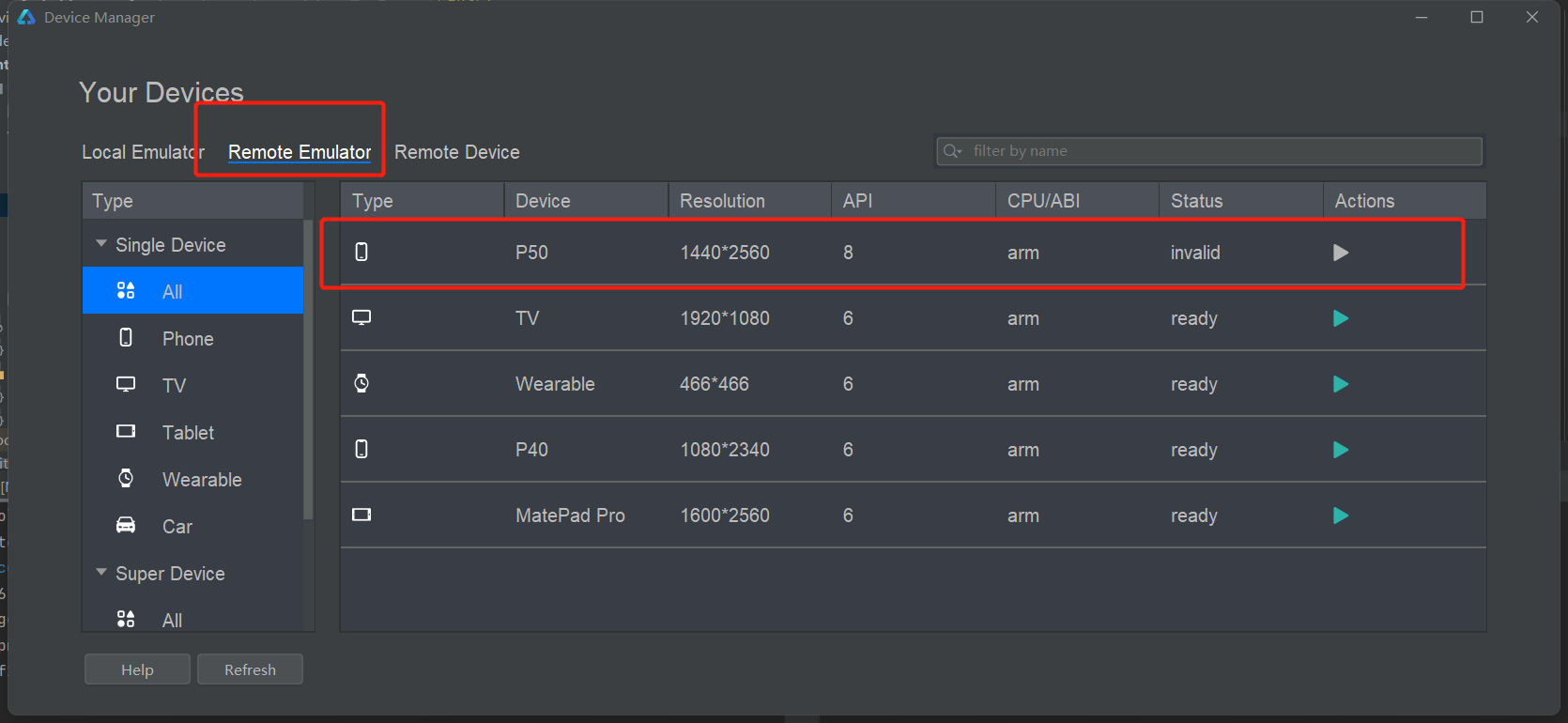## 一、基本需求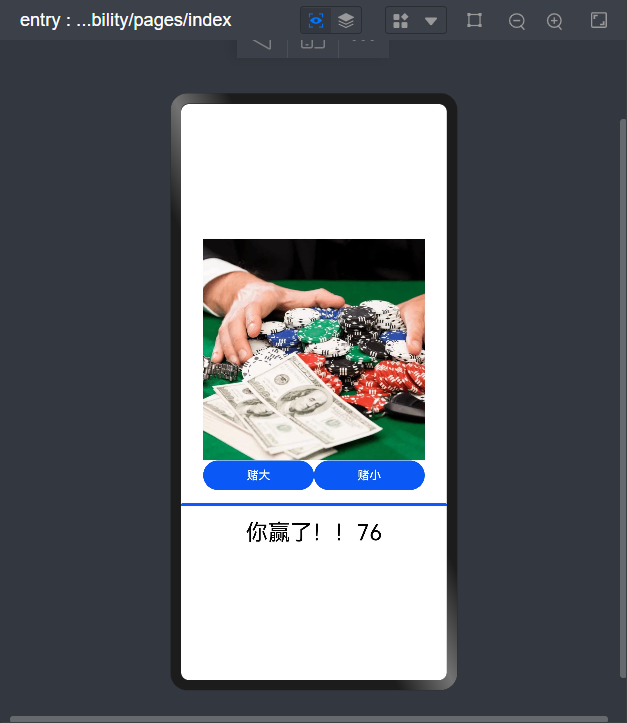# Progress：官方文档

``````interface ProgressInterface {
(options: ProgressOptions): ProgressAttribute;
}

declare interface ProgressOptions {
value: number; // 必须要指定初始进度
total?: number;
style?: ProgressStyle
type?: ProgressType
}
``````

value number - 指定当前进度值。
total number 100 指定进度总长。
type ProgressType ProgressType.Linear 指定进度条样式。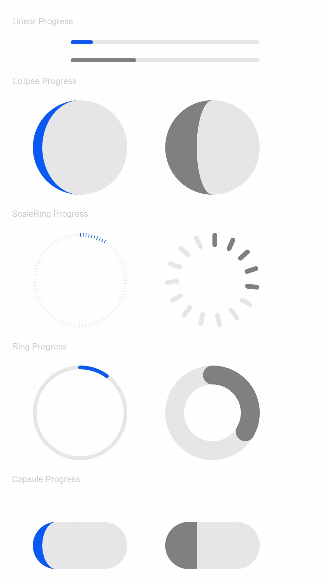# Image

## 权限说明

``````"abilities": [
{
...
"permissions": ["ohos.permission.INTERNET"],
...
}
]
``````
``````interface ImageInterface {
(src: string | PixelMap | Resource): ImageAttribute;
}
src：设置要加载的图片资源，支持从本地、网络和内存中加载图片，简单样例如下：
Image(\$r("app.media.test"))
.width(180)
.height(80)
``````

## 三、UI设计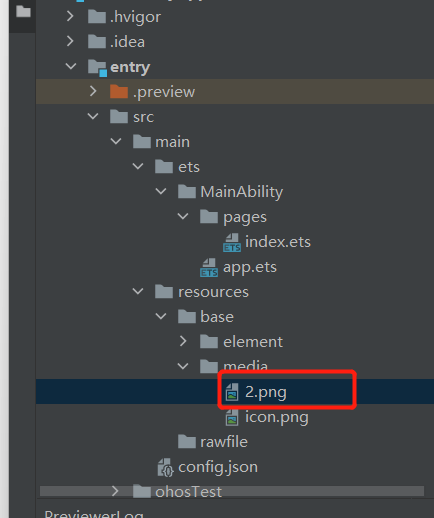``````        Image(\$r("app.media.2"))
.width(300)
.height(300)
```````````` Row() {
Button('赌大')
.width(150)
.onClick(() => {

})
Button('赌小')
.width(150)
.onClick(() => {
})
}
````````````         @State Set_Num: number = 0;
Progress({
value: this.Set_Num,                   // 设置当前进度
total: 100,                  // 设置进度总量
type: ProgressType.Linear    // 设置进度条的样式为条形样式
})
.size({width: '100%', height: 40})
``````## 四、功能设计

``````function sleep(ms){
return new Promise((resolve)=>setTimeout(resolve,ms));
}

async Get_Result()
{
this.Set_Num = 0
for(var i = 0;i<=100;i++)
{
var temple = await sleep(10);
this.Set_Num = i
}
}
``````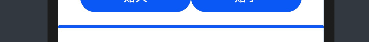``````this.The_Result = Math.ceil(Math.random() * 100 + 1)
``````

``````async Get_Result()
{
this.Set_Num = 0
for(var i = 0;i<=100;i++)
{
var temple = await sleep(10);
this.Set_Num = i
}
this.The_Result = Math.ceil(Math.random() * 100 + 1)
if(this.Flag){
if(this.The_Result<50)
{
this.message = '你输了！！' + this.The_Result.toString()
}else
{
this.message = '你赢了！！'+ this.The_Result.toString()
}

}else
{
if(this.The_Result>=50)
{
this.message = '你输了！！'+ this.The_Result.toString()
}else
{
this.message = '你赢了！！'+ this.The_Result.toString()
}

}
}

``````

``````        Row() {
Button('赌大')
.width(150)
.onClick(() => {
this.Flag = 1
this.Get_Result()

})
Button('赌小')
.width(150)
.onClick(() => {
this.Flag = 0
this.Get_Result()
})
}
``````

## 五、功能演示